# Laplace equation

A homogeneous partial differential equation of the form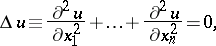(1)

where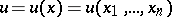is a function ofreal variables. The left-hand side of the Laplace equation is called the Laplace operator acting on. Regular solutions of the Laplace equation of class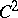in some domainof the Euclidean space,, that is, solutions that have continuous partial derivatives up to the second order in, are called harmonic functions (cf. Harmonic function) in. The Laplace equation is the main representative of second-order partial differential equations of elliptic type, for which fundamental methods of solution of boundary value problems for elliptic equations (cf. Boundary value problem, elliptic equations) have been and are being developed.

Let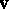be a potential vector field in, that is,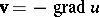, whereis the potential. Since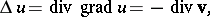the physical meaning of the Laplace equation is that it is satisfied by the potential of any such field in source-free domains. For example, the Laplace equation is satisfied by the gravitational potential of the gravity force in domains free from attracting masses, the potential of an electrostatic field in a domain free from charges, etc. Thus, the Laplace equation expresses the conservation law for a potential field. From this point of view the form (1) of the Laplace equation is obtained by choosing a rectangular Cartesian coordinate system; in other coordinate systems the Laplace operator and the Laplace equation take a different form. In the presence of sources of the field there appears a function proportional to the density of the sources on the right-hand side of (1), and the Laplace equation becomes the Poisson equation. The Laplace equation also arises in many other problems in mathematical physics in which stationary fields are considered, for example, in the study of a stationary temperature distribution, in problems of static elasticity theory, etc.

The following boundary value problems of potential theory are fundamental for the Laplace equation: 1) the Dirichlet problem, or first boundary value problem, in which one looks for a harmonic function that takes given continuous values on the boundary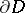of the domain; 2) the Neumann problem, or second boundary value problem, in which one looks for a harmonic functionsuch that its normal derivative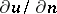takes given continuous values on; and 3) the mixed problem, in which one looks for a harmonic functionthat satisfies a linear relationon the boundary.

In the casethe Laplace equation is closely connected with the theory of analytic functions of a complex variable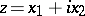, which are characterized by the fact that their real and imaginary parts are conjugate harmonic functions.

The Laplace equation occurs in papers of L. Euler and J. d'Alembert (see , ) in connection with problems of hydromechanics and the first studies of functions of a complex variable. However, it became widely known after the appearance of the papers of P.S. Laplace (see , ) on the theory of the gravitational potential and celestial mechanics.

Equation (1) is sometimes called the scalar Laplace equation, by contrast with the vector Laplace equation(2)

For example, in the case of a vector field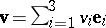, defined in a rectangular Cartesian coordinate system of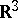, the vector Laplace equation (2) is equivalent to three scalar Laplace equationsfor each of the components,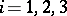. In other coordinate systems the vector Laplace equation is equivalent to a system of three partial differential equations of the second order for the components of the vector field, obtained from (2) after carrying out the operations of vector analysis in the corresponding coordinates (see ).

How to Cite This Entry:
Laplace equation. Encyclopedia of Mathematics. URL: http://encyclopediaofmath.org/index.php?title=Laplace_equation&oldid=33907
This article was adapted from an original article by E.D. Solomentsev (originator), which appeared in Encyclopedia of Mathematics - ISBN 1402006098. See original article